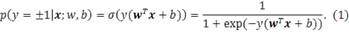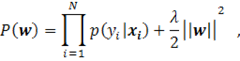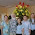## Friday, February 11, 2011

### Logistic Regression in F# using Microsoft Solver Foundation

Logistic regression is a workhorse in data mining. Like decision trees and SVMs, it is a very standard classifier. If you have a labeled data, logistic regression definitely is one of the classifiers that should tried.

In this post, I’d like to show how to implement a logistic regression using Microsoft Solver Foundation in F#. Because we use the optimization in Microsoft Solver Foundation, the core part of a logistic regression contains only 20 – 30 lines of code.

If you are only interested in F# and L-BFGS, but not logistic regression, please only read the second section: L-BFGS solver in Microsoft Solver Foundation.

## Background for logistic regression

Logistic regression model predicts the probability that a data instance x being labeled as yA data instance is a vector with each dimension representing a value for some feature. For example, the Iris flower data set we are going to use in this post has 4 features: Sepal Length, Sepal Width, Petal Length and Petal Width. Each instance has a label y indicating the category. The above model only models binary labels. Logistic regression also has multiple categories version, see [Mitchell]. In this post, we focus on the binary version; however multiple versions should be straightforward once we know how to implement the binary one.

The model contains two parameters weight vector w and offset b, which should be estimated/learned on the training data set. In our derivations below, we will drop the offset b, because we can always add one feature (constant 1) to feature vector x’=[x 1] and hide b into the extended w’=[w b]. Sometimes, a model without the offset parameter could also perform equally well. Because equation (1) measures the probability, we could solve the model parameters by optimizing the joint probability estimated on dataset X.

If we assume that all the instances are independent and identically distributed (i.i.d.). Then the regularized joint probability iswhereis the regularization term for avoiding over-fitting on the training data.

The log version of this likelihood is

We want to find the model parameter w that maximizes this likelihood. P(X) is a concave function with respect to w, so it has a global maximal.

What left is how to optimize this function. BFGS is a Quasi-Newton method that only needs first order gradient while a Newton family method needs second order explicitly. Microsoft Solver Foundation has a limited memory version of BFGS (L-BFGS). (L-BFGS is more popular than BFGS, sometimes people mention BFGS to mean L-BFGS.) There is a memory parameter m in L-BFGS, the space complexity of this algorithm is O(md), where d is the dimension of the variable space. Say, if you want to optimize a function that has 1,000,000 free variables, and set m to 10, then you need only a little more than 1M*10*8/1024^2=76M bytes.

The input for an L-BFGS optimizer is: 1) the function evaluator L(w) and 2) the gradient evaluator:The output is the bestthat gives the maximum function value.

## L-BFGS solver in Microsoft Solver Foundation

Microsoft Solver Foundation already implements an L-BFGS solver. The reader is suggested to read pages 24-27 & 72-75 of MSF-SolverProgrammingPrimer.pdf (on my machine: C:\Program Files\Microsoft Solver Foundation\3.0.1.10599\Documents) for detailed reference. Because the code for optimizing Rosenbrock function is in C#, here I translated it to F#. Note how I translate between delegate type System.Func<> and F# functions.

Btw, I find that using the Microsoft.Solver.Foundatoin.dll in Sho would be more convenient as it packages everything into one DLL.

#r @"C:\Program Files (x86)\Sho 2.0 for .NET 4\packages\Optimizer\Microsoft.Solver.Foundation.dll"
openMicrosoft.SolverFoundation.Common
openMicrosoft.SolverFoundation.Solvers
openMicrosoft.SolverFoundation.Services

letsolverParams = newCompactQuasiNewtonSolverParams()
letsolver = newCompactQuasiNewtonSolver()
let_, vidVaribaleX = solver.AddVariable(null)
let_, vidVaribaleY = solver.AddVariable(null)

//add a row and set it as the goal
let_, vidRow = solver.AddRow(null)

letOriginalRosenbrockFunction =
letf (model:INonlinearModel)  (rowVid:int)  (values:ValuesByIndex)  (newValues:bool) =
Math.Pow(1. - values., 2.) + 100. * (Math.Pow(values. - (values. * values.), 2.))
newSystem.Func<INonlinearModel, int, ValuesByIndex, bool, float> (f)

letf (model:INonlinearModel) (rowVid:int) (values:ValuesByIndex) (newValues:bool) (gradient:ValuesByIndex) =
gradient. <- -2. * (1. - values.) - 400. * values. * (values. - (values. * values.))
gradient. <- 200. * (values. - (values. * values.))
newSystem.Action<INonlinearModel, int, ValuesByIndex, bool, ValuesByIndex> (f)

solver.FunctionEvaluator <- OriginalRosenbrockFunction
solver.Solve(solverParams);

Console.WriteLine(solver.ToString());

and the output:

`> Minimize problemDimensions = 2Variable indexes: 1, 2Starting point = 0, 0Solution quality is: LocalOptimaNumber of iterations performed: 21Number of evaluation calls: 27Finishing point =0.999999997671584, 0.999999995285991Finishing value =5.74844710320033E-18val it : unit = ()> `

## Implementation for logistic regression

We first get a sample data set online:

`module Net =     open System.Net    let fetchUrlSimple (url:string) =         let req = WebRequest.Create(url)         let response = req.GetResponse()        use stream = response.GetResponseStream()        use streamreader = new System.IO.StreamReader(stream)        streamreader.ReadToEnd()     type dataset =     { features: float array array; // (instance = float array) array      mutable labels: int array; //     }    let iris =     let page = Net.fetchUrlSimple @"http://archive.ics.uci.edu/ml/machine-learning-databases/iris/iris.data"    let lines = page.Split([|'\n'|], StringSplitOptions.RemoveEmptyEntries)    let getIrisId (s:string) =             if s = "Iris-setosa" then            2        elif s = "Iris-versicolor" then            -1        else            1    let instances =         lines        |> Array.map (fun line ->             let s = line.Split ','            let features = [| "1.0"; s.; s.; s.; s. |] |> Array.map float // add a dumy 1.0 into the fatures             let label = getIrisId s.            features, label            )        |> Array.filter (fun (_, label) -> label <> 2)            let F, L = Array.unzip instances    {        features = F;        labels = L;    }`

The logistic regression implementation:

`module LogReg =     let mutable lambda = 0.1    let dotProduct (x:float array) (g:ValuesByIndex) =        let mutable dot = 0.0        for i=0 to x.Length-1 do            dot <- dot + x.[i] * g.[i+1]        dot    let sigmoid (x:float array) (y:int) (g:ValuesByIndex) =         let mutable dot = 0.0        for i=0 to x.Length-1 do            dot <- dot + x.[i] * g.[i+1]        let z = (float y) * dot        (* code to deal with extream numbers, but with some issues with l(w) calculation        if z > 30.0 then            1.0        elif z < -30.0 then            0.0        else        *)        1.0 / (1.0 + exp (- z))    let sigmoid2 (x:float array) (y:int) (g:float array) =         let mutable dot = 0.0        for i=0 to x.Length-1 do            dot <- dot + x.[i] * g.[i]        1.0 / (1.0 + exp (- (float y) * dot))            let logregValue(ds:dataset) =         let dim = ds.features..Length        let f (model:INonlinearModel)  (rowVid:int)  (values:ValuesByIndex)  (newValues:bool) =             let mutable L = 0.0                        for i=1 to dim do                L <- L + values.[i]*values.[i]            L <- - (L * lambda / 2.0)                        for i=0 to ds.features.Length-1 do                //L <- L - log (1.0 + exp (- (float ds.labels.[i]) * dotProduct ds.features.[i] values ))                L <- L + log (sigmoid ds.features.[i] ds.labels.[i] values)            // printfn "L = %.10f" L            L                    new System.Func<INonlinearModel, int, ValuesByIndex, bool, float> (f)    let logregGradient(ds:dataset) =         // printfn "gradient calculated"        let dim = ds.features..Length        let f (model:INonlinearModel) (rowVid:int) (values:ValuesByIndex) (newValues:bool) (gradient:ValuesByIndex) =             for j=1 to dim do                 gradient.[j] <- -lambda * values.[j]                        for i=0 to ds.features.Length-1 do                let coef = (1.0 - sigmoid ds.features.[i] ds.labels.[i] values) * (float ds.labels.[i])                for j=1 to dim do                     gradient.[j] <- gradient.[j] + coef * ds.features.[i].[j-1]        new System.Action<INonlinearModel, int, ValuesByIndex, bool, ValuesByIndex> (f)    let makeSolver(ds:dataset) =         // set the solver parameters         let solverParams = new CompactQuasiNewtonSolverParams()        //solverParams.IterationLimit <- 11        //solverParams.Tolerance <- 1e-4        // the memory parameter m, default 17        solverParams.IterationsToRemember <- 10        // solver        let solver = new CompactQuasiNewtonSolver()        let dim = ds.features..Length        // add variables        for i=1 to dim do             solver.AddVariable(null) |> ignore                // add a row and set it as the goal        let _, vidRow = solver.AddRow(null)        solver.AddGoal(vidRow, 0, false) |> ignore        // set funcation evaluator and gradient evaluator        solver.FunctionEvaluator <- logregValue(ds)        solver.GradientEvaluator <- logregGradient(ds)                // solve!        solver.Solve(solverParams) |> ignore                // get the optimized point (w) in the solver        let w = Array.create dim 0.0        for i=1 to dim do            w.[i-1] <- solver.GetValue(i).ToDouble()        w`

Try our logistic regression model on the Iris dataset:

`let sol = LogReg.makeSolver(iris)let nWrongs = ref 0for i=0 to iris.features.Length-1 do    let prob = LogReg.sigmoid2 iris.features.[i] iris.labels.[i] sol    printfn "L = %A sig value = %A label = %A" iris.labels.[i] prob (if prob > 0.5 then "correct" else incr nWrongs; "wrong")`

## Reference

Tom Mitchell, Generative and Discriminative Classifiers: Naive Bayes and Logistic Regression, new book chapter. This chapter gives a good introduction to logistic regression. For a more theoretic comparison, see: Ng & Jordan: on discriminative vs generative classifiers a comparison of logistic regression and naïve bayes.

Thomas P. Minka, A comparison of numerical optimizers for logistic regression.

It is well written, the first page ready gives a concise introduction to logistic regression. The rest of the paper has a lot of discussion on optimization methods. Tom also has a Matlab package.

Galen Andrew and Jianfeng Gao. Scalable training of L1-regularized log-linear models. In Proceedings of the 24th International Conference on Machine Learning (ICML 2007), pp. 33-40, 2007.

L1-regularized logistic regression with an implementation in C++.

libLBFGS(LBFGS in C): http://www.chokkan.org/software/liblbfgs/

## Note

I quite like this post because it combines so many good things together: F#, logistic regression, optimization and playing with sample datasets.

1.Interesting post, thank you. I have meant to try out Solver Foundation for a while, and I think that moment is coming closer now.

2.Thanks.
What would you recommend to show a graph?

3.@ Art,

Microsoft Solver Foundation does not have any plot functionality. You can go to Sho library for the plot.

4.Very nice post, I wish use F# for data minning as soon.

5.This comment has been removed by the author.

6.7.@Chris,

Great to see the OCaml version!!

8.Yes It's a nice post this post is very use full for me

Logistic Service Provider